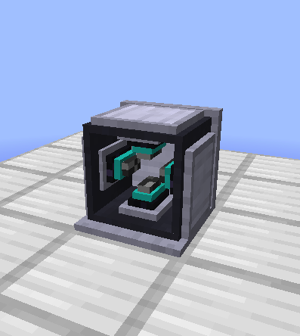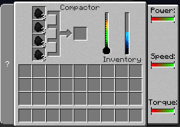## FANDOM

139 PagesA Compactor

(Insert a detailed description of the machine here)

## Rotarycraft Handbook DescriptionEdit

The compactor can compress coal until it becomes anthracite, then prismane, then lonsdaleite, then diamond. Each state will require four of the input items per unit output. All steps require enormous pressures of 550,000kPa and temperatures of greater than 800C to take place. The compactor will gradually heat or cool depending on its surroundings, and pressure is built up by applying high torques.

## RequirementsEdit

Minimum Power:

 Power 524288W Torque 4096 Nm Power Input Back

Power needed to compress coal*

 Power 4,194,304 W Torque 4,194,304 Nm Power Input Back
• To compress coal a massive amount of torque is needed. One method to generate the needed torque is to use a Tier 2 Industrial Coil (240TJ max). Set the Industrial Coil to 1024 rad/s and 4096 Nm, then use 2 CVT units both set to 32:1 and set to torque. This setup will provide enough Torque to achieve the needed pressure to compress coal to diamond. (Note: This processes will take a few minutes to achieve the needed pressure and it will take a few minutes to perform the compression processes. This system will require a lot of energy. It will consume about ~15GJ of energy per hour.
• However, you can get away with less power by setting the coil to 2866 Nm and waiting a fair bit longer (about half an hour) for the pressure to get high enough. See "Tips & Tricks" below for more details on exactly how much power is needed.

## InterfaceEditCompactor GUI

• To be added

## Screwdriver UsageEdit

 Right Click Shift + Right Click

## Duration of Operation FormulaEdit

• Stage 1: Time(in ticks) = 300 - 15 × log₂(speed + 1)
• Stage 2: Time(in ticks) = 600 - 30 × log₂(speed + 1)
• Stage 3: Time(in ticks) = 900 - 45 × log₂(speed + 1)
• Stage 4: Time(in ticks) = 1200 - 60 × log₂(speed + 1)

## Tips & TricksEdit

The Compactor is affected by the ambient pressure, which in turn is affected by height, dimension, and if the compactor is under a fluid. Setting up the compactor in these locations will decrease the pressure (and therefore torque) you have to add to the machine. Here is a list of some default ambient pressures.

• Ambient (Overworld 30 <= y <= 128) = 101kPa
• y < 30 = 126.25kPa
• y > 128 = 80.8kPa
• y > 192 = 64.64kPa
• Ambient (Nether 30 <= y <= 128) = 20,000kPa
• y < 30 = 25,000kPa
• y > 128 = 16,000kPa
• y > 192 = 12,800kPa
• Under Water = 10kPa increase per meter of water (this is added to the ambient)
• Under Lava = 27kPa increase per meter of lava (also added to ambient)

The final pressure the machine achieves will be dimFac*floor(128*log₂(torque)) above ambient pressure; the minimum torques to achieve the 550 MPa required to compress coal in various conditions are noted below:

 Overworld (dimFac=200) Nether (dimFac=600) Deep (y < 30) 2,933,874 Nm 115 Nm Normal (30 <= y <= 128) 2,933,874 Nm 120 Nm Elevated (128 <= y <= 192) 2,933,874 Nm 124 Nm Sky (y > 192) 2,933,874 Nm 128 Nm Deep + 64m of water 2,886,596 Nm 114 Nm Deep + 64m of lava 2,794,314 Nm 114 Nm

Due to the way the calculation works, ambient pressure really only matters whenever you hit multiples of 200 kPa. As such, pressure in the overworld is effectively constant unless you go out of your way to put the machine under lots of fluid.

The Nether appears to be almost unfair due its higher natural pressure, but it's actually rigged since the minimum torque to even run the machine at all is 4,096 Nm. That minimum input torque results in a final pressure of 948,328 kPa, well above the machine's max threshold of 600,000 kPa (past which it explodes).

The required torque is most easily supplied with some power source at 4 MW, but with a shaft junction set to 3:1 it's possible to either "reclaim" 1 MW of power with an industrial coil or use it for something else. Alternatively, the exact power usage can be even more fine-tuned if ElectriCraft is also installed.